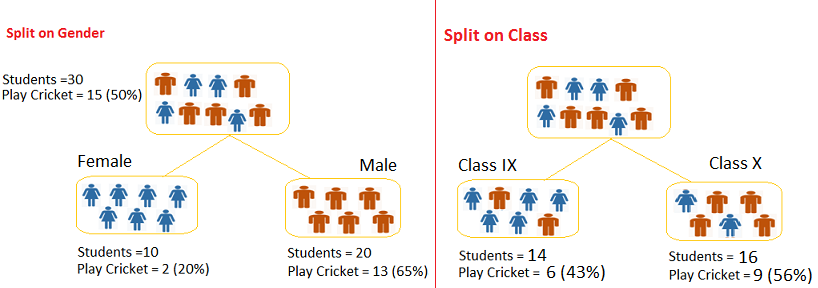# Decision Trees Explained Easily

Decision Trees (DTs) are a non-parametric supervised learning method used for classification and regression. Decision trees learn from data to approximate a sine curve with a set of if-then-else decision rules. The deeper the tree, the more complex the decision rules and the fitter the model.

Decision tree builds classification or regression models in the form of a tree structure. It breaks down a data set into smaller and smaller subsets while at the same time an associated decision tree is incrementally developed. The final result is a tree with decision nodes and leaf nodes. A decision node has two or more branches. Leaf node represents a classification or decision. The topmost decision node in a tree which corresponds to the best predictor called root node. Decision trees can handle both categorical and numerical data.

These are similar to flowcharts.

### How Do Decision Trees Work?

There are several steps involved in the building of a decision tree.

### Splitting

The process of partitioning the data set into subsets. Splits are formed on a particular variableSplitting is done on various factors

### Pruning

The shortening of branches of the tree. Pruning is the process of reducing the size of the tree by turning some branch nodes into leaf nodes, and removing the leaf nodes under the original branch. Pruning is useful because classification trees may fit the training data well, but may do a poor job of classifying new values. A simpler tree often avoids over-fitting.

As you can see, a pruned tree has less nodes and has less sparsity than a unpruned decision tree.

### Tree Selection

The process of finding the smallest tree that fits the data. Usually this is the tree that yields the lowest cross-validated error.

### 1. Entropy

A decision tree is built top-down from a root node and involves partitioning the data into subsets that contain instances with similar values (homogeneous). ID 3 algorithm uses entropy to calculate the homogeneity of a sample. If the sample is completely homogeneous the entropy is zero and if the sample is an equally divided it has entropy of one.

### 2. Information Gain

The information gain is based on the decrease in entropy after a dataset is split on an attribute. Constructing a decision tree is all about finding attribute that returns the highest information gain (i.e., the most homogeneous branches).

Steps Involved

### Step 1:

Calculate entropy of the target.

### Step 2:

The dataset is then split on the different attributes. The entropy for each branch is calculated. Then it is added proportionally, to get total entropy for the split. The resulting entropy is subtracted from the entropy before the split. The result is the Information Gain, or decrease in entropy.

### Step 3:

Choose attribute with the largest information gain as the decision node, divide the dataset by its branches and repeat the same process on every branch.

### Pros:

Computionally cheap to use, easy for humans to understand results and it can deal with irrelevant feaures also

### Cons:

Prone to Overfitting.(It refers to the process when models is trained on training data too well that any noise in testing data can bring negative impacts to performance of model.)

### In Nutshell

A decision tree classifier is just like a flowchart diagram with the terminal nodes representing classification outputs/decisions. Starting with a dataset, you can measure the entropy to find a way to split the set until all the data belonngs to the same class. There are several approaches to decision trees like ID3, C4.5, CART and many more. For splitting nominal valued datasets you can use the ID3 algorithm. You can use matplotlib library to visualize the tree data. Decision Trees are prone to overfitting, thus to avoid overfitting you can prune the decision tree by combining the adjacent nodes that have low information gain.